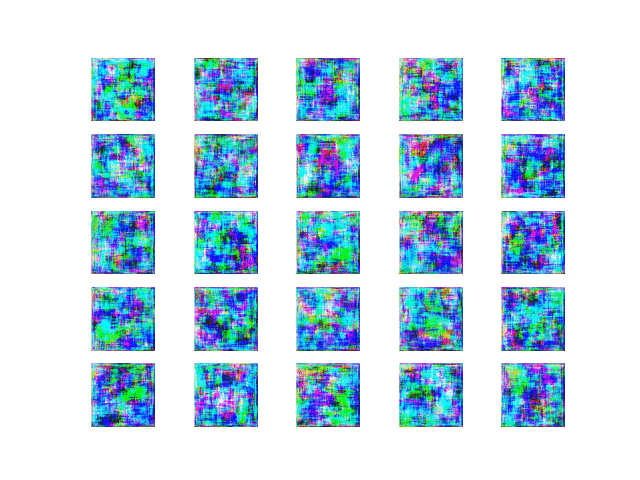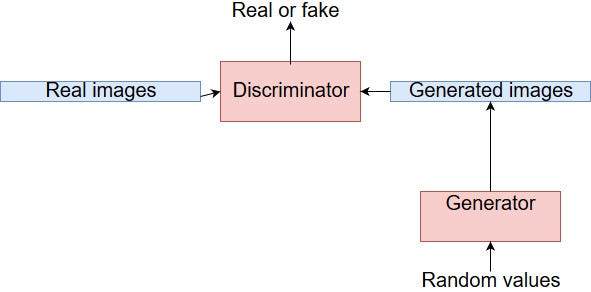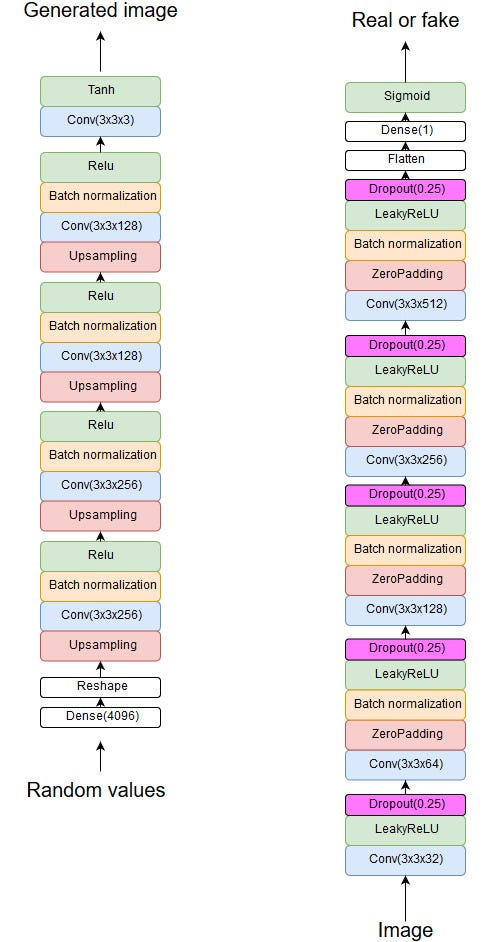Generating Human Faces with KerasThis is the training process in summary.

The idea

I wanted to build something interesting, that is the only way I stay motivated. I had already practiced building several classification algorithms, so I decided that it was the time to build a content generation algorithm.

I looked for interesting datasets on Kaggle and found these two:

Both contain images of human faces. I combined these two into a single folder. It was time to build a human face generator.

Choosing the right network for the task

Two image generation techniques that I hear the most about, are generative adversarial networks (GAN) and LSTM-networks.

First I tried to build an LSTM that could predict pixel values given previous pixels, but it was very slow to train. Then I tried a GAN and it trained much faster. It took less than half an hour to see real results. Blurry faces started appearing. Over time, images got more realistic.

There are many GAN-variants. One that I used is called deep convolutional neural network (DCGAN). What is great about DCGAN is that it uses convolutional layers. Convolutional neural networks are at this moment the best image classification algorithm that exists. So why not use it for image generation too?

Some theory for complete beginners. (If you are already familiar with GANs, skip this part)

Generative adversarial networks were invented by a researcher called Ian Goodfellow and he introduced GANs in 2014.

GANs are very powerful. With the right data, network architecture and hyperparameters you could generate very realistic images.

In the future, some advanced version of GANs or some other content generation algorithm will likely enable us to do cool stuff like:

1. Generate photorealistic video games.
2. Generate movies.
3. Generate 3D designs for new technology (better cars, spaceships, etc)

But how does a GAN work?GAN is not actually one neural network, but two. One of them is the generator. It takes random values as an input and produces an image.

Second is the discriminator. It attempts to determine whether an image is fake or real.

Training a GAN is like an arms race. Generator tries to become as good as possible at fooling the discriminator. Discriminator tries to become as good as possible at separating fake images from the real ones.

This will force both of them to improve. Ideally, this will lead at some point to the following situation:

1. The generator generates images that are for humans indistinguishable from the real ones.
2. Discriminator network reaches an accuracy of 50%. In other words, discriminator cannot separate real and fake ones, so it has to guess each time.

In reality, you would need to have everything right (data, architecture, hyperparameters). GANs are very sensitive to small changes in hyperparameter values. I noticed this effect myself when I was training my GAN.

If you are serious about building a GAN then read this blog post:

Keep Calm and train a GAN. Pitfalls and Tips on training Generative Adversarial Networks

It will explain how you should improve your network in order to get better results.

Architecture of my GANI ended up with this structure after testing the network many times. My computer almost died when I tried to add even more filters to the generator.

In order to understand this architecture, you need to understand convolutional neural networks.

Read this if you are a beginner or need to refresh your knowledge: https://skymind.ai/wiki/convolutional-network

Importing libraries

The first step is to import all the libraries that are needed.

FaceGenerator class

This piece of code initializes some important variables that are needed for training.

image_width, simage_height = size of a generated image in pixels

channels = amount of color channels in the generated image

random_noise_dimension = amount of random values that generator takes as an input

optimizer = optimizer for backpropagation

discriminator = A convolutional neural network that attempts to determine whether an image is fake or real

generator = A convolutional neural network that generates images. Attempts to fool the discriminator.

random_input = A placeholder for random values. We will use it to feed random values into the generator.

generated_image = output from the generator

validity = how well did generator fool the discriminator

combined = generator and discriminator combined into one model. Instead of training generator separately, it is trained through a combined model. This is required in order to backpropagate the loss.

Load the training data into the model

This function takes the name of a folder as an input and returns all images inside that folder as a numpy array. All images are resized to the size that was specified in the __init__ function.

Shape = (amount of images, width, height, channels).

Networks

These two functions define the generator and the discriminator.

Training

Training loop goes as follows:

For each epoch:

1. Select randomly half of the real images to use in this epoch.
2. Create an array of random numbers between 0 and 1. This will be the input of the generator. Shape = (batch_size, self.random_noise_dimension)
3. Generate new images. Amount of generated images is equal to the batch size.
4. Train the discriminator on real and fake images.
5. Calculate the average loss of the discriminator.
6. Train the generator using the combined model.
7. Print loss values.
8. Generate images and save them if the amount of epochs equals to the next interval.

After training:

1. Save the trained model for later use.

Displaying the results

This function creates a 5 * 5 grid of generated images. This grid is saved as a “.png” file.

Generating images after training

This function can be used to generate new images after training.

Conclusion

Training GANs is hard, and when you succeed, the feeling can be very rewarding.

This code can easily be used for other image datasets. Keep in mind that you may need to edit the network architecture and parameters, depending on images that you are trying to generate.